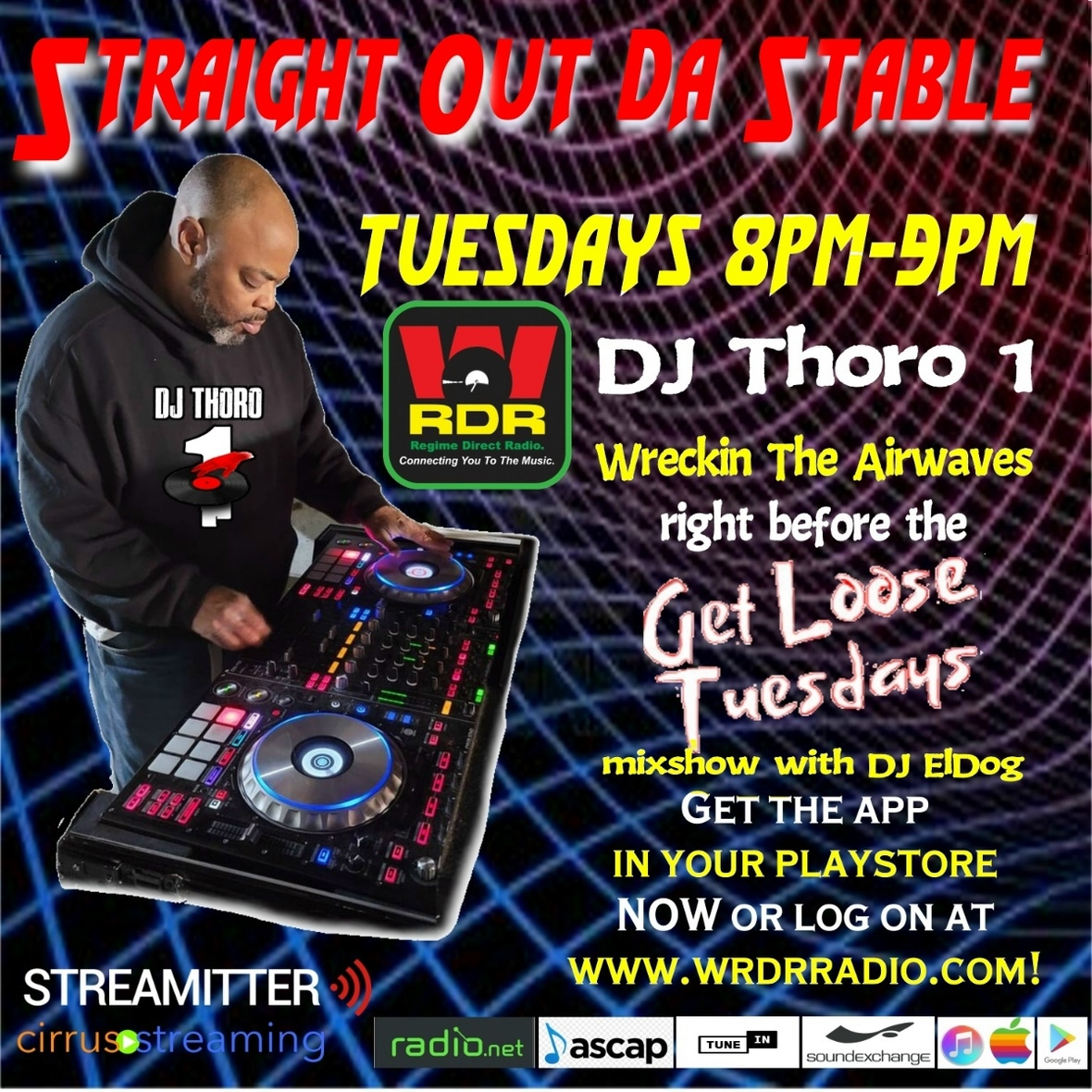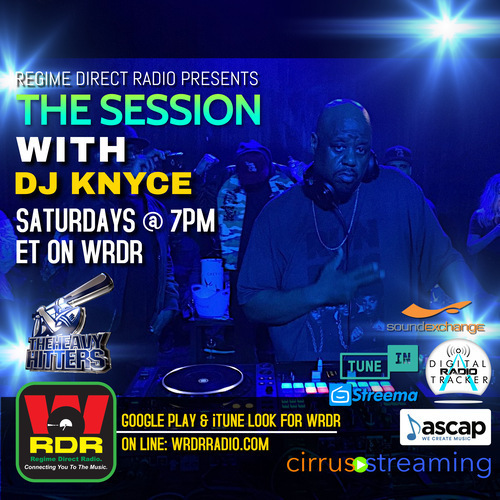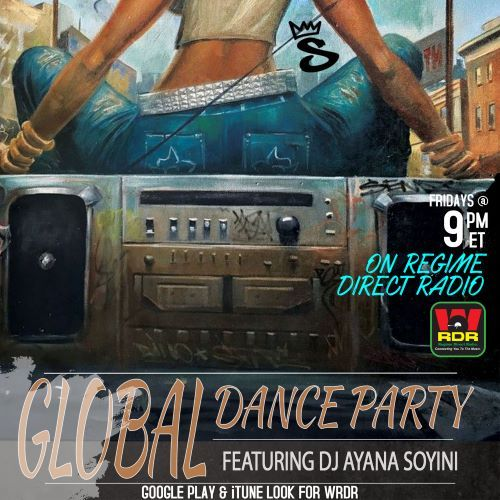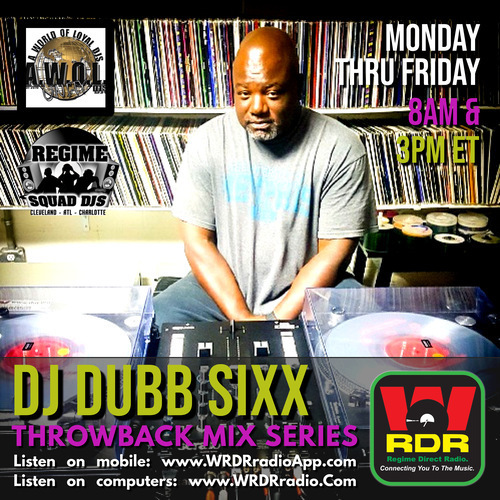# THE MUSIC AND SHOWS ON WRDR ARE X-PLOSIVE!

 table div table+table+table div table{width:100%;padding:0}table div table+table+table div table img{width:96.23%;padding:0;float:none}table div table+table+table div table td{width:100%;padding:0 1.88% 18px}/* styles */table div table+table+table+table div table,table.module-3{width:41.51%;float:right;padding:0}table div table+table+table+table div table a{border:0 none;text-decoration:none}table div table+table+table+table div table img{width:100%!important;border:0 none;text-decoration:none}table div table+table+table+table div table td{width:100%;padding:0 0 20px 20px}/* styles */ The music on WRDR is incredible and the daily line up is “Crazy X-plosive”! WRDR brings you the hottest indie and chart bang’n cuts of Hip & R&B, plus we give you the international flavor of Afrobeats, Dancehall, Urban House Music & Millennial urban talk radio shows. There is no shortage of the urban feel at WRDR with the exciting music with superb sound. This is WRDR | Connecting You To The Music!

# SUNDAYS ON WRDR

 table div table+table+table+table+table+table+table div table{width:100%;padding:0}table div table+table+table+table+table+table+table div table img{width:96.23%;padding:0;float:none}table div table+table+table+table+table+table+table div table td{width:100%;padding:0 1.88% 18px}/* styles */# THE GET IT IN SHOW HOSTED BY DJ CENTIPEDE

Its "The Get It In Show" hosted by DJ Centipede Sundays 3-5 PM ET on WRDR! Listen with a Friend & Tune in!# IT'S R&B GROOVE CONNECTION ON WRDR WITH MusicMan G

It's R&B Groove Connection on WRDR with MusicMan G, Sundays at 6PM ET on WRDR! Ladies he is here, listen in!!!# DJ RAVER WITH THE POP OFF PARTY MIX

It's DJ Raver with The Pop Off Party Mix every Sunday @ 7PM ET. Raver brings the heat with new and Indie music, talk, & wicked beats on the Ones & Twos live! Don't miss The Pop Off Party Mix on WRDR

 table div table+table+table+table+table+table+table+table+table+table+table+table+table div table td,table.module-12{width:100%;padding:0}table div table+table+table+table+table+table+table+table+table+table+table+table+table div table{width:100%;float:none;margin-left:auto;margin-right:auto;padding:0}table div table+table+table+table+table+table+table+table+table+table+table+table+table div table a{border:0 none;text-decoration:none}table div table+table+table+table+table+table+table+table+table+table+table+table+table div table img{width:100%!important;border:0 none;text-decoration:none}/* styles */

# IT’S PSYCHOTIC SUNDAY WITH DJ KRAZI L

Its Psychotic Sunday with DJ Krazi L every other Sunday @ 8PM PM ET on WRDR! Sundays are DOPE on WRDR! Let's get it!# MONDAYS ON WRDR

 table div table+table+table+table+table+table+table+table+table+table+table+table+table+table+table+table+table div table{width:100%;padding:0}table div table+table+table+table+table+table+table+table+table+table+table+table+table+table+table+table+table div table img{width:96.23%;padding:0;float:none}table div table+table+table+table+table+table+table+table+table+table+table+table+table+table+table+table+table div table td{width:100%;padding:0 1.88% 18px}/* styles */# DJ K. NIKKI "MONDAY MADNESS"

It's DJ K Nikki with "Monday Madness" every Monday at 7PM ET on WRDR! She rocks it, listen in!!# DJ BLAC JAC WITH THE CARIBBEAN JUMP OFF

It's DJ Blac Jac with The Caribbean Jump Off Mondays 8-9 PM ET on WRDR! Tune in!

 table div table+table+table+table+table+table+table+table+table+table+table+table+table+table+table+table+table+table+table+table+table div table td,table.module-20{width:100%;padding:0}table div table+table+table+table+table+table+table+table+table+table+table+table+table+table+table+table+table+table+table+table+table div table{width:100%;float:none;margin-left:auto;margin-right:auto;padding:0}table div table+table+table+table+table+table+table+table+table+table+table+table+table+table+table+table+table+table+table+table+table div table a{border:0 none;text-decoration:none}table div table+table+table+table+table+table+table+table+table+table+table+table+table+table+table+table+table+table+table+table+table div table img{width:100%!important;border:0 none;text-decoration:none}/* styles */

# LEX LUGA WITH “THE TRAFIKING HOUR”

It's Lex Luga with "The Trafiking Hour" showcasing new Hip-Hop & R&B and Indie Artist Mondays @ 10PM ET on WRDR! Tune in!# TUESDAYS ON WRDR

 table div table+table+table+table+table+table+table+table+table+table+table+table+table+table+table+table+table+table+table+table+table+table+table+table+table div table{width:100%;padding:0}table div table+table+table+table+table+table+table+table+table+table+table+table+table+table+table+table+table+table+table+table+table+table+table+table+table div table img{width:96.23%;padding:0;float:none}table div table+table+table+table+table+table+table+table+table+table+table+table+table+table+table+table+table+table+table+table+table+table+table+table+table div table td{width:100%;padding:0 1.88% 18px}/* styles */# MILLENNIAL MADNESS WITH DOUG BROWN

It's DJ Doug Brown with his show Millennial Madness every Tuesday @ 7PM ET on WRDR! Listen with a Friends & Tune in!!# DJ THORO 1 WITH STRAIGHT OUT DA STABLE

It's DJ Thoro 1 with Straight Out Da Stable every Tuesday at 8PM on WRDR! DO NOT MISS THIS X-PLOSIVE SHOW!# GET LOOSE TUESDAYS WITH DJ EL DOG

Its Get Loose Tuesdays with DJ El Dog. Tuesdays @ 9PM ET on WRDR! Be there!# THE FLAVA RADIO SHOW WITH BRIA CHERI

 /* styles */ Keeping the airwaves hot is Bria Cheri with The Flava Radio Show every Tuesday at 10PM ET on WRDR! Join her for the X-Clusive interviews, new music with featured artist tracks, celebrity news & gossip. Bria has her finger on the pulse! Be there every Tuesday on WRDR!!!# WEDNESDAYS ON WRDR

 table div table+table+table+table+table+table+table+table+table+table+table+table+table+table+table+table+table+table+table+table+table+table+table+table+table+table+table+table+table+table+table+table+table+table+table+table div table{width:100%;padding:0}table div table+table+table+table+table+table+table+table+table+table+table+table+table+table+table+table+table+table+table+table+table+table+table+table+table+table+table+table+table+table+table+table+table+table+table+table div table img{width:96.23%;padding:0;float:none}table div table+table+table+table+table+table+table+table+table+table+table+table+table+table+table+table+table+table+table+table+table+table+table+table+table+table+table+table+table+table+table+table+table+table+table+table div table td{width:100%;padding:0 1.88% 18px}/* styles */# WATCH YA KNEE CAPS WEDNESDAYS

It's Watch ya knee caps Wednesdays with DJ Short Dawg every single Wednesday at 12:30 PM on WRDR! Be there!# THE SESSION ON WRDR WITH DJ KNYCE

DJ Knyce brings "The Session" Wednesdays at 7PM ET on WRDR! Let's get it! Tune in!!!# VIDEOS FOR THE SOUL BY DJ DWIGHT JONES

WRDR Presents Videos for the Soul by DJ Dwight Jones. Wednesdays 8-10 PM ET on Twitch.Tv. Tune in!# WEDNESDAY HUMP DAY PARTY WITH DJ EMCEE FREE

It's The Wednesday Night Hump Day House Party on WRDR with DJ Emcee Free at 9 PM ET. Tune in! See you there!!!# THURSDAYS ON WRDR

 table div table+table+table+table+table+table+table+table+table+table+table+table+table+table+table+table+table+table+table+table+table+table+table+table+table+table+table+table+table+table+table+table+table+table+table+table+table+table+table+table+table+table+table+table+table+table div table{width:100%;padding:0}table div table+table+table+table+table+table+table+table+table+table+table+table+table+table+table+table+table+table+table+table+table+table+table+table+table+table+table+table+table+table+table+table+table+table+table+table+table+table+table+table+table+table+table+table+table+table div table img{width:96.23%;padding:0;float:none}table div table+table+table+table+table+table+table+table+table+table+table+table+table+table+table+table+table+table+table+table+table+table+table+table+table+table+table+table+table+table+table+table+table+table+table+table+table+table+table+table+table+table+table+table+table+table div table td{width:100%;padding:0 1.88% 18px}/* styles */# THE OLD SKOOL VS. NU SKOOL MIX SHOW WITH DJ DIZZI DEE

Thursdays Dizzi Dee "BATTLES it OUT" with the Old Skool Vs. Nu Skool Mix Show @ 7PM ET! Tune in!!!# DJ RED BOTTOM IN THE RED ROOM

It's DJ Red Bottom "In The Red Room". Thursdays @ 8PM ET on WRDR! Tune in!!# THURSDAY VIBEZ ON WRDR WITH DJ XL

It's Thursday Vibez on WRDR with DJ XL at 9PM ET. Rock with him! See you there!!!# FRIDAYS ON WRDR

 table div table+table+table+table+table+table+table+table+table+table+table+table+table+table+table+table+table+table+table+table+table+table+table+table+table+table+table+table+table+table+table+table+table+table+table+table+table+table+table+table+table+table+table+table+table+table+table+table+table+table+table+table+table+table div table{width:100%;padding:0}table div table+table+table+table+table+table+table+table+table+table+table+table+table+table+table+table+table+table+table+table+table+table+table+table+table+table+table+table+table+table+table+table+table+table+table+table+table+table+table+table+table+table+table+table+table+table+table+table+table+table+table+table+table+table div table img{width:96.23%;padding:0;float:none}table div table+table+table+table+table+table+table+table+table+table+table+table+table+table+table+table+table+table+table+table+table+table+table+table+table+table+table+table+table+table+table+table+table+table+table+table+table+table+table+table+table+table+table+table+table+table+table+table+table+table+table+table+table+table div table td{width:100%;padding:0 1.88% 18px}/* styles */# DJ ROCKY JR WITH THE OVERDRIVE MIX

It's DJ Rocky JR with the 'OVERDRIVE MIX" every Friday at 7PM ET on WRDR! Get your "OVERDRIVE" on, listen in!!!!# GLOBAL FEQUENCY WITH GRANDMIXER GMS

Check out Global Frequency with Grandmixer GMS Fridays at 5PM PT/8PM ET on WRDR! Tune in!# THE GLOBAL DANCE PARTY FEATURING AYANA SOYINI

It's DJ Ayana Soyini from Soyini Sound taking you all over the world playing the best in Afrobeats, Soulful House, and Soca Fridays @ 9PM ET. It's a Global Dance Party on WRDR. Be there for DJ Ayana Soyini!# MILLENNIAL ANONYMOUS PODCAST WITH LEISE WINNY

The MillennialZ Anonymous show is a safe space for millennial issues such as student loans, relationships, politics, self-care and more. MillennialZ Anonymous is led by Leise Winny, a millennial with an attitude. Leise is causing ruckus by forcing millennials to think outside the box with challenging topics, and guests.

Tune in every Friday at 10 PM ET on WRDR for Leise Winny hosting MillennialZ Anonymous!# SATURDAYS ON WRDR

 table div table+table+table+table+table+table+table+table+table+table+table+table+table+table+table+table+table+table+table+table+table+table+table+table+table+table+table+table+table+table+table+table+table+table+table+table+table+table+table+table+table+table+table+table+table+table+table+table+table+table+table+table+table+table+table+table+table+table+table+table+table+table+table+table div table{width:100%;padding:0}table div table+table+table+table+table+table+table+table+table+table+table+table+table+table+table+table+table+table+table+table+table+table+table+table+table+table+table+table+table+table+table+table+table+table+table+table+table+table+table+table+table+table+table+table+table+table+table+table+table+table+table+table+table+table+table+table+table+table+table+table+table+table+table+table div table img{width:96.23%;padding:0;float:none}table div table+table+table+table+table+table+table+table+table+table+table+table+table+table+table+table+table+table+table+table+table+table+table+table+table+table+table+table+table+table+table+table+table+table+table+table+table+table+table+table+table+table+table+table+table+table+table+table+table+table+table+table+table+table+table+table+table+table+table+table+table+table+table+table div table td{width:100%;padding:0 1.88% 18px}/* styles */# COOL TLC WITH THE WORLD FAMOUS MELTDOWN SHOW

It's Cool TLC with The World Famous Meltdown Show every Sunday @ 12PM ET. Cool TLC delivers a great radio hour of fantastic music, talk radio, and excitement, all combined! Tuesdays will not be the same. Tune into Cool TLC with The World Famous Meltdown Show on WRDR!# THE POWER HOUR WITH DJ COCO-Z

It's The Power Hour with DJ CoCo-Z every Saturday @ 1PM ET on WRDR! Don't miss it! CoCo-Z has you on lock! Tune in!# THE DAY PARTY ON WRDR HOSTED BY THE REGIME SQUAD DJS

It's "The Day Party" hosted by the Regime Squad DJs every week bringing you the hottest music to party on a mid-day Saturday @ 3PM ET on WRDR. Tune in!# ROCK'N THE TABLES TOUGH WITH DJ KUE ONE

It's DJ Kue One "Rock'N The Tables Tough" every Saturday @ 8PM ET on WRDR! Listen with a Friends & Tune in!# CITY BEATZ RADIO WITH DJ DUBB SIXX

It's Dubb Sixx with City Beatz Radio every Saturday @ 8PM CT/9PM ET on WRDR! Tunein and catch incredible DJ mixes by Dubb Sixx and special guest appearances by nationwide DJ and artist.# WEEKDAYS M-F ON WRDR

 table div table+table+table+table+table+table+table+table+table+table+table+table+table+table+table+table+table+table+table+table+table+table+table+table+table+table+table+table+table+table+table+table+table+table+table+table+table+table+table+table+table+table+table+table+table+table+table+table+table+table+table+table+table+table+table+table+table+table+table+table+table+table+table+table+table+table+table+table+table+table+table+table+table+table+table+table div table{width:100%;padding:0}table div table+table+table+table+table+table+table+table+table+table+table+table+table+table+table+table+table+table+table+table+table+table+table+table+table+table+table+table+table+table+table+table+table+table+table+table+table+table+table+table+table+table+table+table+table+table+table+table+table+table+table+table+table+table+table+table+table+table+table+table+table+table+table+table+table+table+table+table+table+table+table+table+table+table+table+table div table img{width:96.23%;padding:0;float:none}table div table+table+table+table+table+table+table+table+table+table+table+table+table+table+table+table+table+table+table+table+table+table+table+table+table+table+table+table+table+table+table+table+table+table+table+table+table+table+table+table+table+table+table+table+table+table+table+table+table+table+table+table+table+table+table+table+table+table+table+table+table+table+table+table+table+table+table+table+table+table+table+table+table+table+table+table div table td{width:100%;padding:0 1.88% 18px}/* styles */# THE WRDR POWER MIXES BY DUBB SIXX

DJ Dubb Sixx crushes the US East Coast @ 8AM & 3PM ET with his Power Mixes that catches the "Old Skool Throwback Flava". Catch him every single work day!# THE WRDR MID-DAY MIX SHOW BY DJ CLASSY B

Need that East Coast USA lunch break and ride home to be dope? Then DJ Classy B is here to take you through a real journey. Check out Classy B every day at 12 noon & 5PM ET here on WRDR# THE MILLENNIAL REVOLUTION RADIO SHOW

The Millennial Revolution Radio Show is bringing the musical revolution to Millennial “ear waves”. Hosted by Leise Winny of Millennialz Anonymous Podcast, who's bringing Cardi B's Drip with Queen B's M.AF.I.A. to NICKI'S revenge for the perfect Millennial listening experience.

“The Revolution Will Not Be Televised” but it will be on WRDR Monday through Friday at 6PM ET. Tune In!!!!table div table+table+table+table+table+table+table+table+table+table+table+table+table+table+table+table+table+table+table+table+table+table+table+table+table+table+table+table+table+table+table+table+table+table+table+table+table+table+table+table+table+table+table+table+table+table+table+table+table+table+table+table+table+table+table+table+table+table+table+table+table+table+table+table+table+table+table+table+table+table+table+table+table+table+table+table+table+table+table+table+table+table+table div table{width:100%;padding:0}table div table+table+table+table+table+table+table+table+table+table+table+table+table+table+table+table+table+table+table+table+table+table+table+table+table+table+table+table+table+table+table+table+table+table+table+table+table+table+table+table+table+table+table+table+table+table+table+table+table+table+table+table+table+table+table+table+table+table+table+table+table+table+table+table+table+table+table+table+table+table+table+table+table+table+table+table+table+table+table+table+table+table+table div table img{width:96.23%;padding:0;float:none}table div table+table+table+table+table+table+table+table+table+table+table+table+table+table+table+table+table+table+table+table+table+table+table+table+table+table+table+table+table+table+table+table+table+table+table+table+table+table+table+table+table+table+table+table+table+table+table+table+table+table+table+table+table+table+table+table+table+table+table+table+table+table+table+table+table+table+table+table+table+table+table+table+table+table+table+table+table+table+table+table+table+table+table div table td{width:100%;padding:0 1.88% 18px}/* styles */table.module-83{width:99.62%;padding:0}table div table+table+table+table+table+table+table+table+table+table+table+table+table+table+table+table+table+table+table+table+table+table+table+table+table+table+table+table+table+table+table+table+table+table+table+table+table+table+table+table+table+table+table+table+table+table+table+table+table+table+table+table+table+table+table+table+table+table+table+table+table+table+table+table+table+table+table+table+table+table+table+table+table+table+table+table+table+table+table+table+table+table+table+table div table{width:99.62%;float:none;margin-left:auto;margin-right:auto;padding:0}table div table+table+table+table+table+table+table+table+table+table+table+table+table+table+table+table+table+table+table+table+table+table+table+table+table+table+table+table+table+table+table+table+table+table+table+table+table+table+table+table+table+table+table+table+table+table+table+table+table+table+table+table+table+table+table+table+table+table+table+table+table+table+table+table+table+table+table+table+table+table+table+table+table+table+table+table+table+table+table+table+table+table+table+table div table a{border:0 none;text-decoration:none}table div table+table+table+table+table+table+table+table+table+table+table+table+table+table+table+table+table+table+table+table+table+table+table+table+table+table+table+table+table+table+table+table+table+table+table+table+table+table+table+table+table+table+table+table+table+table+table+table+table+table+table+table+table+table+table+table+table+table+table+table+table+table+table+table+table+table+table+table+table+table+table+table+table+table+table+table+table+table+table+table+table+table+table+table div table img{width:100%!important;border:0 none;text-decoration:none}table div table+table+table+table+table+table+table+table+table+table+table+table+table+table+table+table+table+table+table+table+table+table+table+table+table+table+table+table+table+table+table+table+table+table+table+table+table+table+table+table+table+table+table+table+table+table+table+table+table+table+table+table+table+table+table+table+table+table+table+table+table+table+table+table+table+table+table+table+table+table+table+table+table+table+table+table+table+table+table+table+table+table+table+table div table td{width:100%;padding:0}/* styles */
 /* styles */ Artist, would you like your track featured on WRDR? Contact WRDR HERE for available and promotional packages that will fit your needs.
 table div table+table+table+table+table+table+table+table+table+table+table+table+table+table+table+table+table+table+table+table+table+table+table+table+table+table+table+table+table+table+table+table+table+table+table+table+table+table+table+table+table+table+table+table+table+table+table+table+table+table+table+table+table+table+table+table+table+table+table+table+table+table+table+table+table+table+table+table+table+table+table+table+table+table+table+table+table+table+table+table+table+table+table+table+table+table div table{width:100%;padding:0}table div table+table+table+table+table+table+table+table+table+table+table+table+table+table+table+table+table+table+table+table+table+table+table+table+table+table+table+table+table+table+table+table+table+table+table+table+table+table+table+table+table+table+table+table+table+table+table+table+table+table+table+table+table+table+table+table+table+table+table+table+table+table+table+table+table+table+table+table+table+table+table+table+table+table+table+table+table+table+table+table+table+table+table+table+table+table div table img{width:96.23%;padding:0;float:none}table div table+table+table+table+table+table+table+table+table+table+table+table+table+table+table+table+table+table+table+table+table+table+table+table+table+table+table+table+table+table+table+table+table+table+table+table+table+table+table+table+table+table+table+table+table+table+table+table+table+table+table+table+table+table+table+table+table+table+table+table+table+table+table+table+table+table+table+table+table+table+table+table+table+table+table+table+table+table+table+table+table+table+table+table+table+table div table td{width:100%;padding:0 1.88% 18px}/* styles *//* styles */ Tune into WRDR to hear the hottest blend of Hip-Hop & R&B featuring Indie & Major artist, and world renowned Urban talk radio shows! This is WRDR | Connecting You To The Music
 table div table+table+table+table+table+table+table+table+table+table+table+table+table+table+table+table+table+table+table+table+table+table+table+table+table+table+table+table+table+table+table+table+table+table+table+table+table+table+table+table+table+table+table+table+table+table+table+table+table+table+table+table+table+table+table+table+table+table+table+table+table+table+table+table+table+table+table+table+table+table+table+table+table+table+table+table+table+table+table+table+table+table+table+table+table+table+table+table+table div table{width:100%;padding:0}table div table+table+table+table+table+table+table+table+table+table+table+table+table+table+table+table+table+table+table+table+table+table+table+table+table+table+table+table+table+table+table+table+table+table+table+table+table+table+table+table+table+table+table+table+table+table+table+table+table+table+table+table+table+table+table+table+table+table+table+table+table+table+table+table+table+table+table+table+table+table+table+table+table+table+table+table+table+table+table+table+table+table+table+table+table+table+table+table+table div table img{width:96.23%;padding:0;float:none}table div table+table+table+table+table+table+table+table+table+table+table+table+table+table+table+table+table+table+table+table+table+table+table+table+table+table+table+table+table+table+table+table+table+table+table+table+table+table+table+table+table+table+table+table+table+table+table+table+table+table+table+table+table+table+table+table+table+table+table+table+table+table+table+table+table+table+table+table+table+table+table+table+table+table+table+table+table+table+table+table+table+table+table+table+table+table+table+table+table div table td{width:100%;padding:0 1.88% 18px}/* styles */Like   Tweet   +1   in